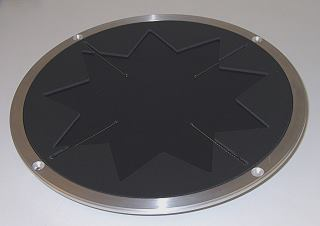# Manger

## Tweeter## Manger W05/1,2.2.8

order no. mg-msw-sw4- not available

20 cm full range driver with high quality cone. The magnet system is based on Neodymium technology.

• frequency range = 80-35000 Hz
• resonance frequency fs = 88 Hz
• impedance R = 4 Ohm
• sound pressure level SPL = 94 dB (2,83V; 1m)
• DC resistance Re = 4,2 Ohm
• force factor BL = 3,1 N/A
• voice coil inductance L = 3,7 mH
• effective piston radiating area Sd = 220 cm2
• equivalent volume of compliance Vas = 15 l
• total Q factor Qts = 0,88 (Qms=2,94, Qes=1,25)
• voice coil diameter = 70 mm
• maximum peak linear excursion vibration xlin = +/- 3,5 mm
• mounting diameter d = 192,5 mm
• overall diameter d = 211 mm
• mounting depth (not countersunk) t = 22 mm
PDF data sheet## Manger W04/1,2.2.8

order no. mg-msw-al4- not available

20 cm full range driver with high quality cone. The magnet system is based on Neodymium technology.

• frequency range = 80-35000 Hz
• resonance frequency fs = 88 Hz
• impedance R = 4 Ohm
• sound pressure level SPL = 94 dB (2,83V; 1m)
• DC resistance Re = 4,2 Ohm
• force factor BL = 3,1 N/A
• voice coil inductance L = 3,7 mH
• effective piston radiating area Sd = 220 cm2
• equivalent volume of compliance Vas = 15 l
• total Q factor Qts = 0,88 (Qms=2,94, Qes=1,25)
• voice coil diameter = 70 mm
• maximum peak linear excursion vibration xlin = +/- 3,5 mm
• mounting diameter d = 192,5 mm
• overall diameter d = 211 mm
• mounting depth (not countersunk) t = 22 mm
PDF data sheet## Manger W05/1,2.2.16

order no. mg-msw-sw8- not available

20 cm full range driver with high quality cone. The magnet system is based on Neodymium technology.

• frequency range = 80-35000 Hz
• resonance frequency fs = 88 Hz
• impedance R = 8 Ohm
• sound pressure level SPL = 91 dB (2,83V; 1m)
• DC resistance Re = 7,2 Ohm
• force factor BL = 4,31 N/A
• voice coil inductance L = 7,44 mH
• effective piston radiating area Sd = 220 cm2
• equivalent volume of compliance Vas = 15 l
• total Q factor Qts = 0,88 (Qms=2,94, Qes=1,25)
• voice coil diameter = 70 mm
• maximum peak linear excursion vibration xlin = +/- 3,5 mm
• mounting diameter d = 192,5 mm
• overall diameter d = 211 mm
• mounting depth (not countersunk) t = 22 mm
PDF data sheet## Manger W04/1,2.2.16

order no. mg-msw-al8- not available

20 cm full range driver with high quality cone. The magnet system is based on Neodymium technology.

• frequency range = 80-35000 Hz
• resonance frequency fs = 88 Hz
• impedance R = 8 Ohm
• sound pressure level SPL = 91 dB (2,83V; 1m)
• DC resistance Re = 7,2 Ohm
• force factor BL = 4,31 N/A
• voice coil inductance L = 7,44 mH
• effective piston radiating area Sd = 220 cm2
• equivalent volume of compliance Vas = 15 l
• total Q factor Qts = 0,88 (Qms=2,94, Qes=1,25)
• voice coil diameter = 70 mm
• maximum peak linear excursion vibration xlin = +/- 3,5 mm
• mounting diameter d = 192,5 mm
• overall diameter d = 211 mm
• mounting depth (not countersunk) t = 22 mm
PDF data sheet

The cabinet volume calculations are executed considering an external resistance of 0.3 Ohm. We are using, if no other data is known or available, the information supplied by the manufacturer.

Please note: the frequency response doesn't say much about the cut-off frequency inside the cabinet! Therefore, we mostly give you 2 examples with dimensioning considerations and an indication, how low the loudspeaker will reproduce.

Home